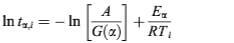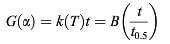# Oxidation kinetics and mechanisms of a 2D C/C composite (2)

The kinetic equation of common type of solid-state decomposition is based on a single-step kinetic equation in terms of the reaction model and the Arrhenius parameters.

Dα/dt=k(T)f(α)                       (1)

Where α is the degree of transformation, t is the time, k(T) is the rate constant depending on temperature, T is the absolute temperature and f(α) is a function depending on the reaction mechanism. The reaction model may take various forms, some of which are shown in Table 1. Eq.(1) is often used in its integral form, which for isothermal conditions becomes

G(α)=k(T)t                          (2)

Where G(α) is the integrated form of the reaction model. The temperature dependence of the rate constant is usually described by the Arrhenius equation:

K(T)=Aexp(-E/RT)                     (3)

Where A and E are the Arrhenius parameters and R is the gas constant. Under isothermal conditions, we may combine Eqs.(2) and (3) to getEq.(4)-     (4)

By the Eq.(4), Eα values can be calculated at progressive degrees of conversion without any assumption about the reaction model. The model-free method can reflect changes in the reaction mechanism and kinetics with the temperature and the extent of conversion, and succeeds in the complex process involving two or more stages.

Equation (5) is taken the natural logarithm of Eq.(2):

In G(α)=In k(T)+In t                (5)

Substituting a particular reaction model into Eq.(5) results in evaluating the corresponding rate constant, which is found from the slope of the plot of G(α) versus t. For each reaction model selected, the rate constants are evaluated at several temperatures and the Arrhenius parameters are evaluated using the Arrhenius equation in its logarithmic form:

In k(T)=InA-E/RT                   (6)

Correlation coefficient (r) is used as a parameter for choosing the model giving best fit. The model-fitting method succeeds in the process controlled by only a mechanism.

In 1966, Sharp et al. Have shown that an approach based on a reduced-time scale facilitates comparison of experimental data with theoretical models. The theoretical equation was expressed in the form:Eq.(7)-  (7)

Where t0.5 is the time at which α=0.5 and B is a calculable constant which depends on the form of G(α). Experimental data can be expressed using reduced time (t/t0.5) by a single curve, which can be compared directly with plots of data calculated from theoretical equations. When the α-t/t0.5 curve of experiment fit plot of theoretical equation, the model of the theoretical equation is the controlling mechanism of this experimental process. Well-known calculated data of reduced time t/t0.5 for the most commonly used solid-state reaction equations are presented in table 2.

### CONTACT US

CFC CARBON CO., LTD/ HTMAGROUP
ADD: Yizhuang Economic Development Zone, Beijing 100176, China.
Fax: +86 10 80828912
Website: www.cfccarbon.com
Email: potter@cfccarbon.com
Marketing center: +86-18618169878
Human Resources: +86-15313026852